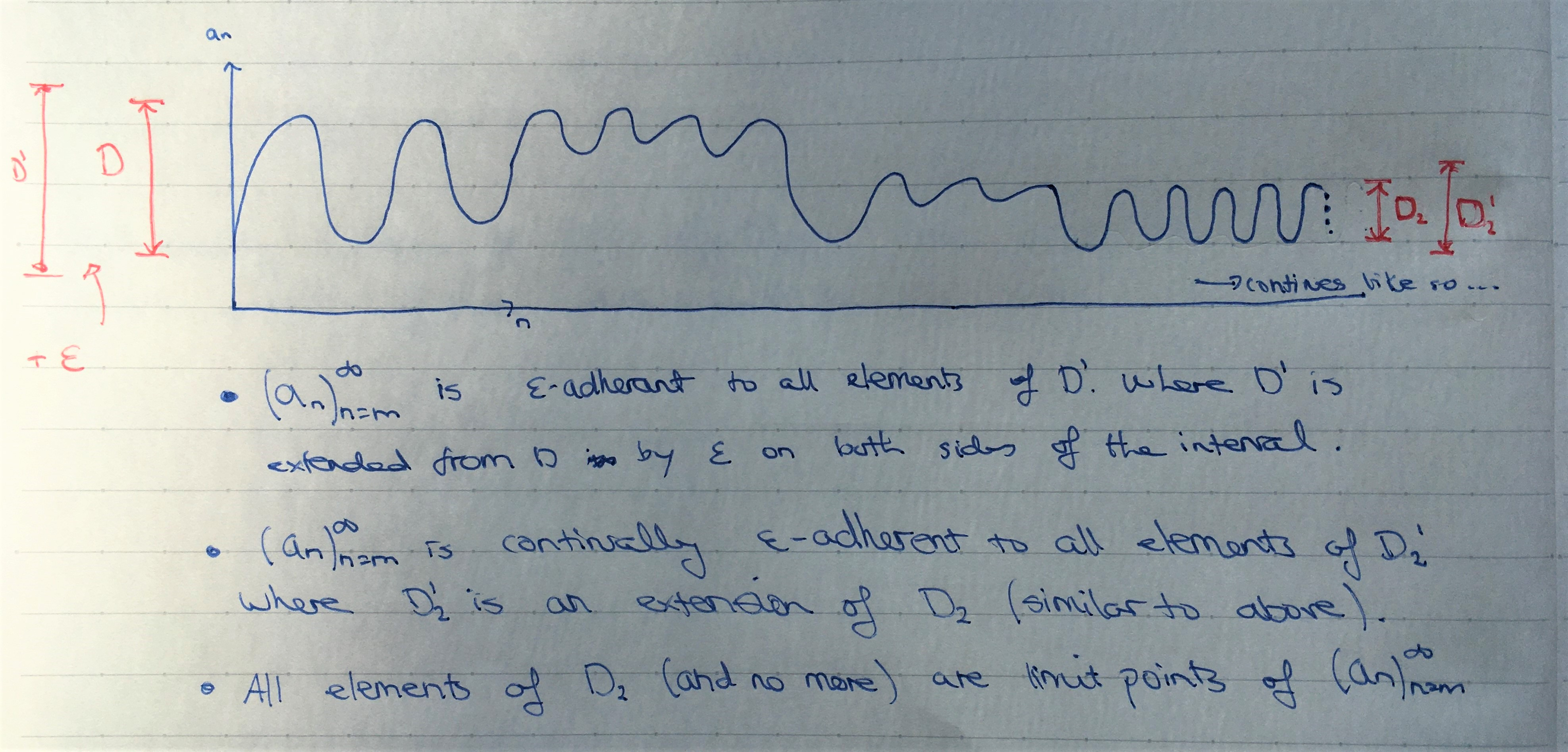Show Question
Math and science::Analysis::Tao::06. Limits of sequences

# Limit points

Let $$(a_n)_{n=m}^{\infty}$$ be a sequence of real numbers, let x be a real number, and let $$\epsilon > 0$$ be a real number. We say that $$x$$ is ε-adherent to $$(a_n)_{n=m}^{\infty}$$ iff there exists an $$n \ge m$$ such that $$a_n$$ is ε-close to x. We say that $$x$$ is continually ε-adherent to $$(a_n)_{n=m}^{\infty}$$ if it is ε-adherent to $$(a_n)_{n=N}^{\infty}$$ for every $$N \ge m$$. We say that $$x$$ is a limit point or adherent point of $$(a_n)_{n=m}^{\infty}$$ if it is continually ε-adherent to $$(a_n)_{n=m}^{\infty}$$ for every $$\epsilon > 0$$

How are limit points different to limits?

The definition is motivated by a sequence such as:

$1.1, -1.01, 1.001, -1.0001, 1.00001,...$

The sequence does not converge, but half the time it is getting closer to to 1, and half the time it is getting closer to -1. We wish to name and describe properties of these numbers which are not limits of the squence, but have some qualities of being limits.

How are limit points different to limits?
In the case of limits, ε-close-ness requires all elements of a sequence to be within a certain distance of a number. For limit points, ε-adherance only requires a single element of the sequence to be within a certain distance of a number. To continue the comparison, eventually ε-close-ness requires some $$N \ge m$$ such that $$(a_n)_{n=N}^{\infty}$$ is ε-close to something, whereas continual ε-adherance requires $$(a_n)_{n=N}^{\infty}$$ to be ε-adherant for all $$N \ge m$$. So in the first case we have a  $$\exists x\, \forall y\, (y \ge x) \supset close(target, y)$$ predicate and in the latter case we have a  $$\forall x\, \exists y\, (y \ge x) \supset close(target, y)$$.

To add to the comparison, it is case that limits are limit points. And if a limit exists, then it is the only limit point. (Proposition 6.4.5)

### ExampleThe above picture uses the verb 'adherant' the wrong way around. Correct: all elements of D' are ε-adherant to the sequence.